# Week 10 Lecture 2: Finite Differences for PDEs

## Rahman notes:

Consider the heat equation problem in the theory lecture: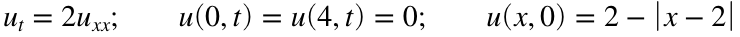We can plot the initial condition
x = [0:0.1:4];
y = 2 - abs(x-2);
plot(x,y,'linewidth', 4)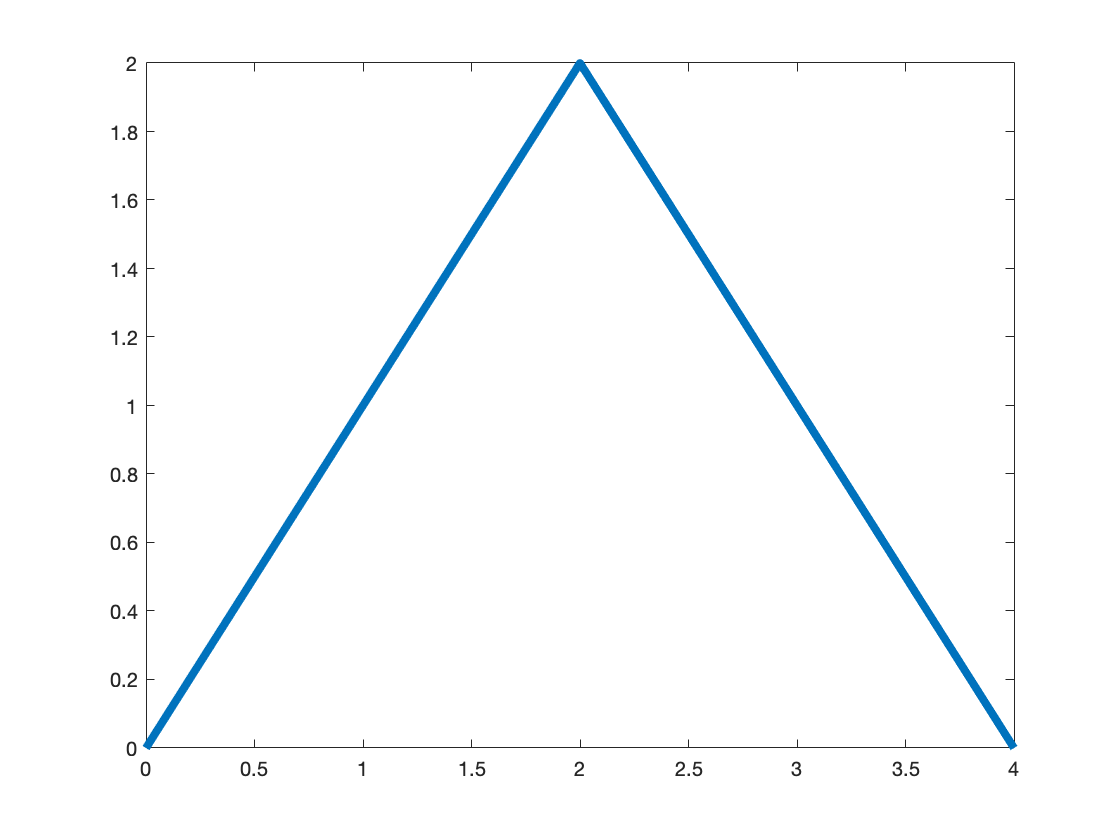Now lets use the Crank-Nicolson scheme (the gold standard for finite differences for the heat equation) to solve this. We saw in the theory lecture that our finite difference scheme simplifies to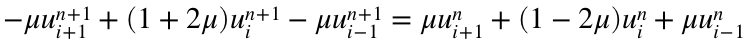this indicates we have a tridiagonal system on both sides of the equation, but since we know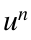we simply do the matrix multiplication to give us a vector on the right hand side. For more detail take a look at the theory lecture.
dt = 0.01;
dx = 0.01;
x = [0:dx:4];
X = length(x);
k = 2;
u = 2 - abs(x-2);
u = u';
mu = k*dt/(2*dx^2);
u_a = 0; u_b = 0; %Dirichlet boundary conditions
main_diag = (1+2*mu)*ones(1,X-2);
second_diag = -mu*ones(1, X-3);
A = diag(main_diag) + diag(second_diag,1) + diag(second_diag, -1);
main_diag = (1-2*mu)*ones(1,X-2);
B = diag(main_diag) - diag(second_diag,1) - diag(second_diag, -1);
plot(x,u)
axis([0 4 0 2])
pause(0.1)
hold on
for t = dt:dt:1
b = B*u(2:end-1);
b(1) = b(1) + mu*u_a;
b(end) = b(end) + mu*u_b;
u(2:end-1) = A\b;
plot(x,u)
axis([0 4 0 2])
pause(0.1)
end
hold off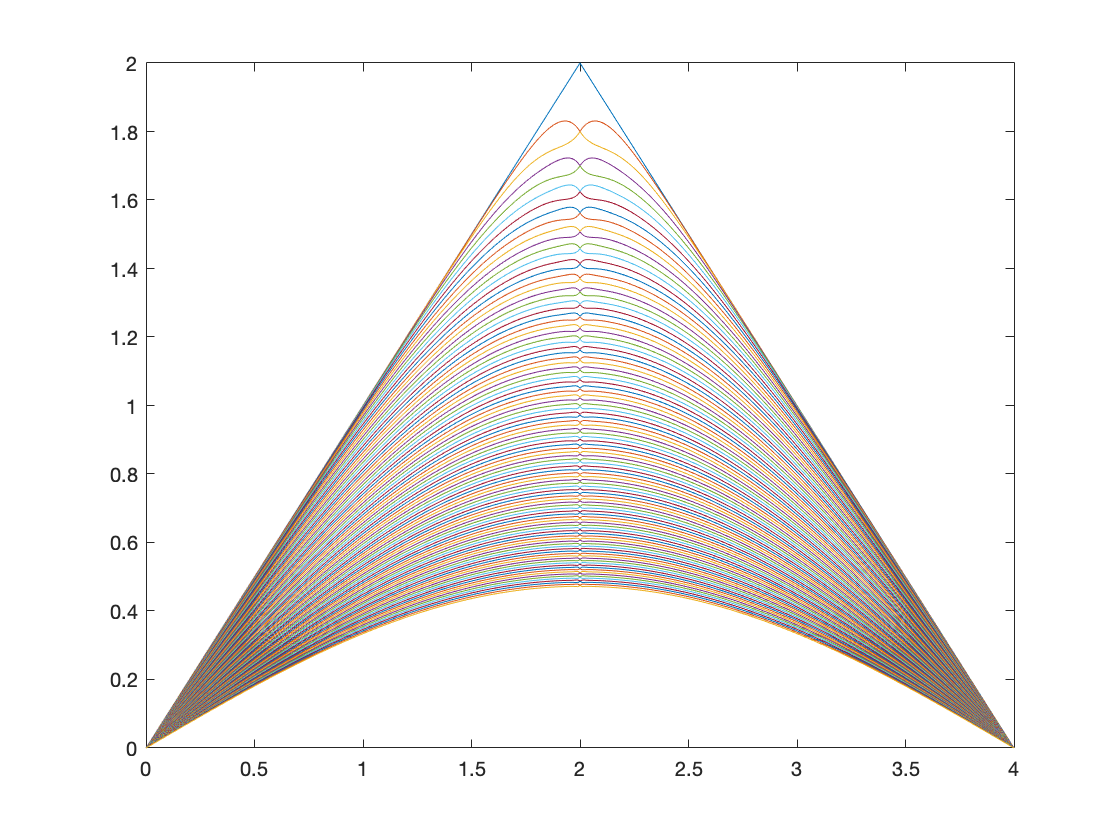Lets try it with an initial condition that doesn't have a kink.
dt = 0.01;
dx = 0.01;
x = [0:dx:4];
X = length(x);
k = 2;
u = 2 - (x-2).^2;
u = u';
mu = k*dt/(2*dx^2);
u_a = 0; u_b = 0; %Dirichlet boundary conditions
main_diag = (1+2*mu)*ones(1,X-2);
second_diag = -mu*ones(1, X-3);
A = diag(main_diag) + diag(second_diag,1) + diag(second_diag, -1);
main_diag = (1-2*mu)*ones(1,X-2);
B = diag(main_diag) - diag(second_diag,1) - diag(second_diag, -1);
plot(x,u)
axis([0 4 0 2])
pause(0.1)
hold on
for t = dt:dt:1
b = B*u(2:end-1);
b(1) = b(1) + mu*u_a;
b(end) = b(end) + mu*u_b;
u(2:end-1) = A\b;
plot(x,u)
axis([0 4 0 2])
pause(0.1)
end
hold off